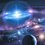# "Depressed Cubic" Equations

Scipione del Ferro solved the "Depressed Cubic" equations.

Tartaglia claimed he solved it(But it is just a copyright infringement)

Having a bet with Antonio Fior in solving this type of equations, he took a long time solving all 30 questions.

Problem is that this type of equations are really hard to solve. View the full method in solving "Depressed Cubic" equations online.

I'm going to introduce a more layman way of solving this type of equations.

${ x }^{ 3 }+mx=n$

Factorise out $x$ from left hand side of the equation and find out the factors for n.

Substitute in the factors and make sure both sides of the equation matches. Solve for $x$

Note that this approach only works when the polynomial equation has an integer root. It is a result of (but not equivalent to) the rational root theorem.Note by Luke Zhang
6 years, 3 months ago

This discussion board is a place to discuss our Daily Challenges and the math and science related to those challenges. Explanations are more than just a solution — they should explain the steps and thinking strategies that you used to obtain the solution. Comments should further the discussion of math and science.

When posting on Brilliant:

• Use the emojis to react to an explanation, whether you're congratulating a job well done , or just really confused .
• Ask specific questions about the challenge or the steps in somebody's explanation. Well-posed questions can add a lot to the discussion, but posting "I don't understand!" doesn't help anyone.
• Try to contribute something new to the discussion, whether it is an extension, generalization or other idea related to the challenge.
• Stay on topic — we're all here to learn more about math and science, not to hear about your favorite get-rich-quick scheme or current world events.

MarkdownAppears as
*italics* or _italics_ italics
**bold** or __bold__ bold
- bulleted- list
• bulleted
• list
1. numbered2. list
1. numbered
2. list
Note: you must add a full line of space before and after lists for them to show up correctly
paragraph 1paragraph 2

paragraph 1

paragraph 2

[example link](https://brilliant.org)example link
> This is a quote
This is a quote
    # I indented these lines
# 4 spaces, and now they show
# up as a code block.

print "hello world"
# I indented these lines
# 4 spaces, and now they show
# up as a code block.

print "hello world"
MathAppears as
Remember to wrap math in $$ ... $$ or $ ... $ to ensure proper formatting.
2 \times 3 $2 \times 3$
2^{34} $2^{34}$
a_{i-1} $a_{i-1}$
\frac{2}{3} $\frac{2}{3}$
\sqrt{2} $\sqrt{2}$
\sum_{i=1}^3 $\sum_{i=1}^3$
\sin \theta $\sin \theta$
\boxed{123} $\boxed{123}$

## Comments

Sort by:

Top Newest

How do you solve the "simplest" case $x^3 + x = 1$?

Your method only works if there is an integer solution.

Staff - 6 years, 3 months ago

Log in to reply

Yes. Only for integers.

- 6 years, 3 months ago

Log in to reply

×

Problem Loading...

Note Loading...

Set Loading...